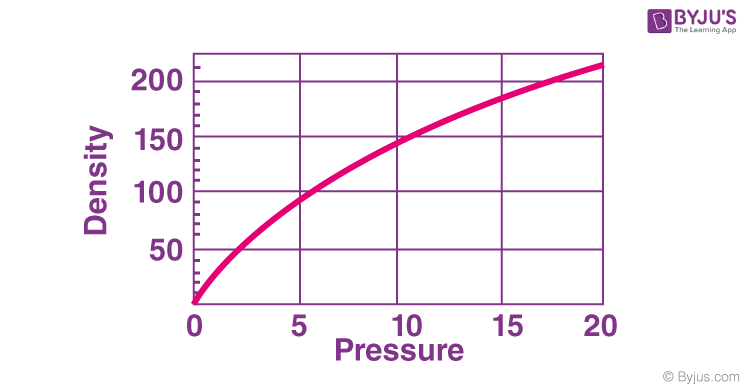# Relation Between Pressure And Density

The pressure is the measure of force acting on a unit area. Density is the measure of how closely any given entity is packed, or it is the ratio of the mass of the entity to its volume. The relation between pressure and density is direct. Change in pressure will be reflected in a change in density and vice-versa.

## Pressure and Density

The pressure and density relationship for ideal gases and fluids is mathematically written as-

 Formula Terms SI units For ideal gas $$P=\rho RT$$ P is the pressure of the ideal gas pascal or Pa R is the universal gas constant R=8.31 J/mole/K0 T is the temperature of the ideal gas Kelvin or K0 $$\rho$$ is the density of the ideal gas. Kg/m3 For fluids $$P=\frac{\rho Vg}{Ag_{c}}$$ P is the pressure of the fluid pascal or Pa or bar $$\rho$$ is the density of the fluid lbm/ft3 V is the volume ft3 A is the area ft2 m is the mass lbm g is the acceleration due to gravity ft/sec2 gc is the gravity constant 32.17lbm-ft/lbf-sec2

## Pressure and Density RelationshipThe pressure and density relation are direct. That is, the pressure is directly proportional to density. Which means that –

• When pressure increases, density increases.
• When the pressure decreases, density decreases.
• When density increases, pressure increases.
• When density decreases, the pressure decreases.

## Pressure and Density Equation

Deriving pressure and density equation is very important to understand the concept. Below is the derivation of pressure and density relation for the ideal gas as well as for fluids.

### Equation of state ideal gas

In thermodynamics, the relation between pressure and density is expressed through equation of states for ideal gases. Consider an ideal gas with

• Pressure P
• Volume V
• Density $$\rho$$
• Temperature T
• Universal gas constant R
• Number of moles n

Applying Boyle’s law and Charles and Gay-Lussac law we get-

• Boyle’s law: For a given mass, at a constant temperature, the pressure times volume is constant. PV = C1
• Charles and Gay-Lussac law: For a given mass, at constant pressure, the volume is directly proportional to the temperature. V = C2T

Combining both we get-

$$\frac{PV}{T}=nR$$ $$\Rightarrow PV=nRT$$

Dividing both sides by mass m we get-

$$\Rightarrow \frac{PV}{m}=\frac{nRT}{m}$$———–(1)

Here, Specific volume(v) can be defined as the ratio of volume to its mass. That is $$v=\frac{Volume}{Mass}=\frac{V}{m}=\frac{1}{\rho }$$

Substituting specific volume in equation(1) we get-

$$\Rightarrow Pv=\frac{nRT}{M}$$

Or

Pv = RT

Or

$$P=\frac{RT}{v}$$ $$\Rightarrow P=\rho RT$$

### Pressure Equation Fluids

In thermodynamics, the pressure of a fluid is given by-

$$P=\frac{F}{A}$$

Here, Force F = mg and Area A = Agc

$$P=\frac{mg}{Ag_{c}}$$

Density $$\rho =\frac{m}{V}\;\;\;\Rightarrow m=\rho V$$

Thus, we get-

$$P=\frac{\rho Vg}{Ag_{c}}$$

Hope you understood the relation between pressure and density in fluid mechanics and thermodynamics.

Physics Related Topics:

Stay tuned with BYJU’S for more such interesting articles. Also, register to “BYJU’S – The Learning App” for loads of interactive, engaging Physics-related videos and an unlimited academic assist.

Test Your Knowledge On Relation Between Pressure And Density!